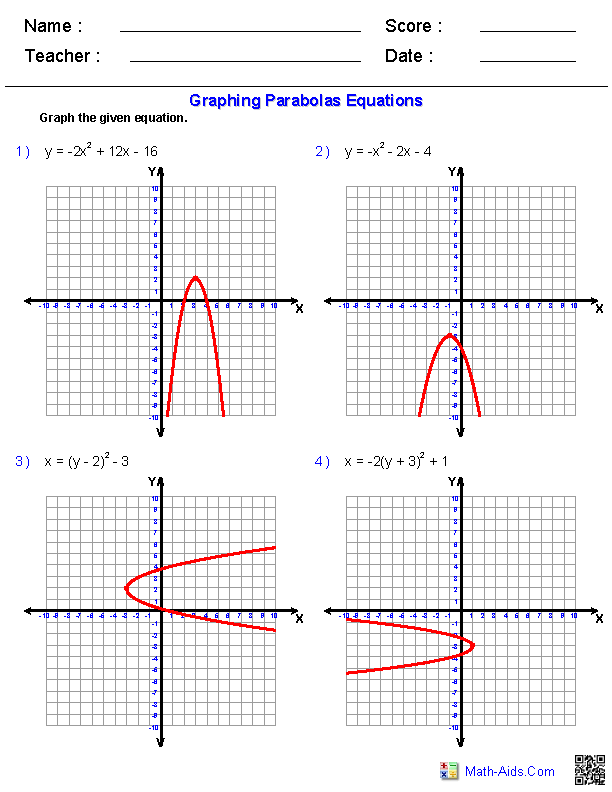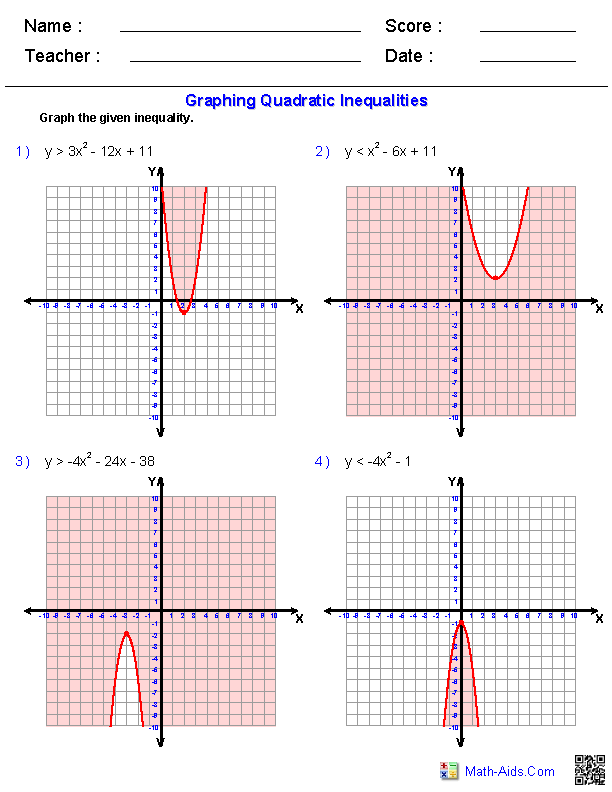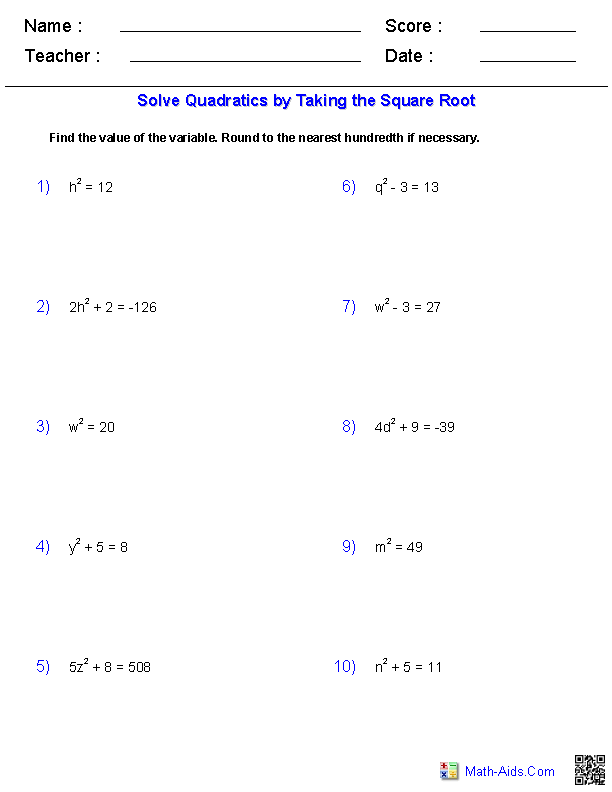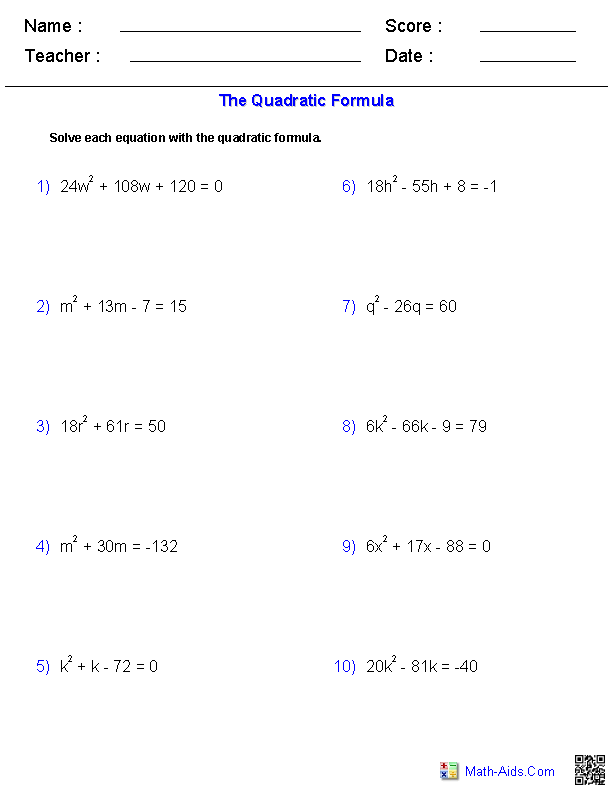#Algebra 1 Worksheets

Here is a graphic preview for all of the Quadratic Functions Worksheets. You can select different variables to customize these Quadratic Functions Worksheets for your needs. The Quadratic Functions Worksheets are randomly created and will never repeat so you have an endless supply of quality Quadratic Functions Worksheets to use in the classroom or at home. We have graphing quadratic functions, graphing quadratic inequalities, completing the square. We also have several solving quadratic equations by taking the square roots, factoring, with the quadratic formula, and by completing the square.

These Quadratic Functions Worksheets are a good resource for students in the 5th Grade through the 8th Grade.

Click the image to be taken to that Quadratic Functions Worksheets.##### Solving Quadratic EquationsBy Taking Square Roots##### Solving Quadratic EquationsBy Completing the SquareRecommended Videos

## Detailed Description for All Quadratic Functions Worksheets

This Algebra 1 - Quadratic Functions Worksheets will produce problems for practicing graphing quadratic function from their equations. These Quadratic Functions Worksheets are a good resource for students in the 5th Grade through the 8th Grade.

This Algebra 1 - Quadratic Functions Worksheet produces problems for graphing quadratic inequalities. You can select the magnitude of the "a" term and the direction in which the parabola opens. These Quadratic Functions Worksheets are a good resource for students in the 5th Grade through the 8th Grade.

Complete the Square Worksheets
These Algebra 1 - Quadratic Functions Worksheets produces problems for completing the square. You may select what type of "b" term you want to use. These Quadratic Functions Worksheets are a good resource for students in the 5th Grade through the 8th Grade.

Taking the Square Roots Worksheets
These Algebra 1 - Quadratic Functions Worksheets produces problems for solving quadratic equations by taking the square root. You may select the difficulty of the problems you want to use. These Quadratic Functions Worksheets are a good resource for students in the 5th Grade through the 8th Grade.

Solving Quadratic Equations by Factoring Worksheets
These Algebra 1 - Quadratic Functions Worksheets produces problems for solving quadratic equations by factoring. You may select the difficulty of the problems you want to use. These Quadratic Functions Worksheets are a good resource for students in the 5th Grade through the 8th Grade.